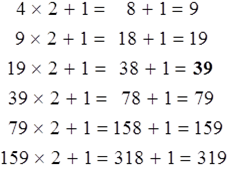# Sequence and Series - Online Test

Q1. Direction: What should come in place of question mark (?) in the following number series?
24, 536, 487, 703, 678, ?
Explaination / Solution:So, 742 will come at the place of question mark.

Q2. Direction: What will come in place of the question mark (?) in the following number series?
18, 27, 49, 84, 132, (?)
Explaination / Solution:So, ? = 193

Q3. Directions: What will come in place of the question mark (?) in each of the following number series?
33, 43, 65, 99, 145, (?)
Explaination / Solution:So, ? = 203

Q4. Direction: What will come in place of the question mark (?) in the following number series?
7, 11, 23, 51, 103, (?)
Explaination / Solution:

The series is
7 + (41) = 11
11 + (43) = 23
23 + (47) = 51
51 + (413) = 103
103 + (421) = 187
So, ? = 103 + (421) = 187

Q5. Directions: What will come in place of the question mark (?) in each of the following number series?
5, 3, 6, ?, 64.75
Explaination / Solution:

5*0.5 + 0.5 = 3
3*1.5 + 1.5 = 6
6*2.5 + 2.5 = 17.5
17.5*3.5 + 3.5 = 64.75
So, 17.5 will come at the place of question mark.

Q6. Directions: What should come in place of question mark (?) in the following number series ?

8, 9, 20, 63, 256, 1285, ?
Explaination / Solution:Q7. Direction: What will come in place of the question mark (?) in the following number series?
1015, 508, 255, 129, 66.5, ?, 20.875
Explaination / Solution:Q8. Direction: What will come in place of the question mark (?) in the following number series?
3, 6, 12, 24, 48, 96, ?
Explaination / Solution:

The pattern is:
3 × 2 = 6
6 × 2 = 12
12 × 2 = 24
24 × 2 = 48
48 × 2 = 96
96 × 2 = 192

Hence Option A is correct

Q9. Directions: What should come in place of the question mark (?) in the following number series?
2, 16, 112, 672, 3360, 13440 , ?
Explaination / Solution:Q10. Directions: What should come in place of the question mark (?) in the following number series?
4, 9, 19, ? , 79, 159, 319
Explaination / Solution: